# NEET Chemistry Exam 2019 year Question Papers and Answer keys

46. The number of sigma (σ) and π(π) bonds in pent-2-en-4-yne is-

(1) 10 σ bonds and 3π bonds

(2) 8 σ bonds and 5π bonds

(3) 11 σ bonds and 2π bonds

(4) 13 σ bonds and no π bonds

Chemistry Notes

Physics Notes

Biology Notes

47.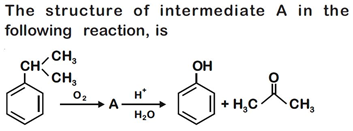(a)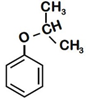(b)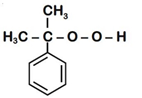(c)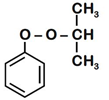(d)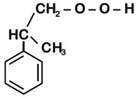48. The correct structure of tribromooctaoxide is

(a)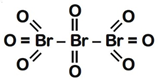(b)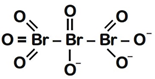(c)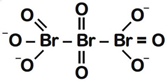(d)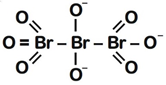49. 4d, 5p, 5f and 6p orbitals are arranged in the order of decreasing energy. The correct option is

(1) 5f > 6p > 5p > 4d                 (2) 6p > 5f > 5p > 4d

(3) 6p > 5f > 4d > 5p                 (4) 5f > 6p > 4d > 5p

Chemistry Notes

Physics Notes

Biology Notes

50.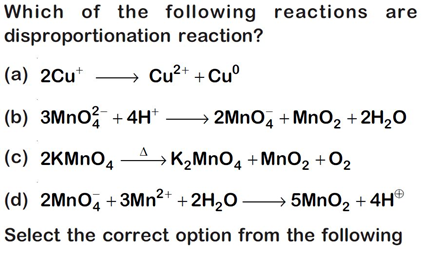(1) (a) and (b) only                    (2) (a), (b) and (c)

(3) (a), (c) and (d)                     (4) (a) and (d) only

51. Under isothermal condition, a gas at 300 K expands from 0.1 L to 0.25 L against a constant external pressure of 2 bar. The work done by the gas is

(Given that 1 L bar = 100 J)

(1) –30 J                (2) 5 kJ

(3) 25 J                  (4) 30 J

Chemistry Notes

Physics Notes

Biology Notes

52. Among the following, the one that is not a green house gas is

(1) Nitrous oxide             (2) Methane

(3) Ozone                        (4) Sulphur dioxide

53.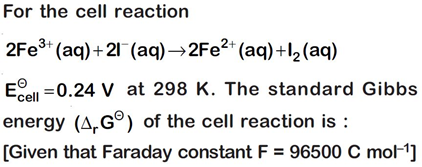(1) – 46.32 kJ mol–1                  (2) – 23.16 kJ mol–1

(3) 46.32 kJ mol–1                     (4) 23.16 kJ mol–1

54. Enzymes that utilize ATP in phosphate transfer require an alkaline earth metal (M) as the cofactor. M is :

(1) Be                    (2) Mg

(3) Ca                    (4) Sr

55.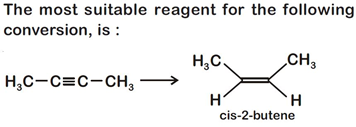(1) Na/liquid NH3                       (2) H2, Pd/C, quinoline

(3) Zn/HCl                                 (4) Hg2+/H+, H2O

Chemistry Notes

Physics Notes

Biology Notes

56. Which is the correct thermal stability order for H2E

(E = O, S, Se, Te and Po)?

(1) H2S < H2O < H2Se < H2Te < H2Po

(2) H2O < H2S < H2Se < H2Te < H2Po

(3) H2Po < H2Te < H2Se < H2S < H2O

(4) H2Se < H2Te < H2Po < H2O < H2S

57. Which of the following is incorrect statement?

(1) PbF4 is covalent in nature

(2) SiCl4 is easily hydrolysed

(3) GeX4 (X = F, Cl, Br, I) is more stable thanGeX2

(4) SnF4 is ionic in nature

58. Match the following :

(a) Pure nitrogen              (i) Chlorine

(b) Haber process            (ii) Sulphuric acid

(c) Contact process          (iii)Ammonia

(d) Deacon’s process       (iv) Sodium azide or Barium azide

Which of the following is the correct option?

(1) (a) – (i) (b) – (ii)       (c) – (iii)       (d) – (iv)

(2) (a) – (ii) (b) – (iv)      (c) – (i)         (d) – (iii)

(3) (a) – (iii)           (b) – (iv)      (c) – (ii)        (d) – (i)

(4) (a) – (iv)           (b) – (iii)      (c) – (ii)        (d) – (i)

59. Which of the following diatomic molecular species has only π bonds according to Molecular Orbital Theory?

(1) O2

(2) N2

(3) C2

(4) Be2

Chemistry Notes

Physics Notes

Biology Notes

60. For the second period elements the correct increasing order of first ionisation enthalpy is:

(1) Li < Be < B < C < N < O < F < Ne

(2) Li < B < Be < C < O < N < F < Ne

(3) Li < B < Be < C < N < O < F < Ne

(4) Li < Be < B < C < O < N < F < Ne

(1) Nylon-6,6        (2) Nylon-2-Nylon 6

(3) Nylon-6           (4) Buna-S

62. pH of a saturated solution of Ca(OH)2 is 9. The solubility product (Ksp) of Ca(OH)2 is:

(1) 0.5 × 10–15                  (2) 0.25 × 10–10

(3) 0.125 × 10–15             (4) 0.5 × 10–10

Chemistry Notes